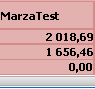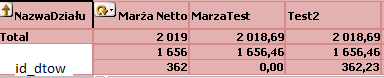# QlikView App Development

Discussion Board for collaboration related to QlikView App Development.

New Contributor III

## Problem with sum total

Hello, I 've got problem bcs my expression is:

=(num(if( id_dtow<>45,(sum(if(OperacjeRachunki=16777234  and StatusRach=0 and mod(Atrybut,2)=0,NettoSprzedazRachunki-NettoZakupuRachunki,0))

+sum(if(OperacjeRachunki=16777238  and StatusRach=0 and mod(Atrybut,2)=0,NettoSprzedazRachunki-NettoZakupuRachunki,0))

+sum(if(OperacjeRachunki=33554450  and StatusRach=0 and mod(Atrybut,2)=0,NettoSprzedazRachunki-NettoZakupuRachunki,0))

+sum(if(OperacjeRachunki=33554454  and StatusRach=0 and mod(Atrybut,2)=0,NettoSprzedazRachunki-NettoZakupuRachunki,0))

+sum(if(OperacjeRachunki=67108882 and StatusRach=0 and mod(Atrybut,2)=0,NettoSprzedazRachunki-NettoZakupuRachunki,0))

//+sum(if(OperacjeRachunki=67108882 and id_tow<>45 and StatusRach=0 and mod(Atrybut,2)=0,NettoSprzedazRachunki-NettoZakupuRachunki,0))

+sum(if(OperacjeRachunki=67108886  and StatusRach=0 and mod(Atrybut,2)=0,-NettoSprzedazRachunki+NettoZakupuRachunki,0))

+sum(if(OperacjeRachunki=486539282  and StatusRach=0 and mod(Atrybut,2)=0,NettoSprzedazRachunki-NettoZakupuRachunki,0))

+sum(if(OperacjeRachunki=486539286  and StatusRach=0 and mod(Atrybut,2)=0,-NettoSprzedazRachunki+NettoZakupuRachunki,0))

//+sum(if(OperacjeRachunki=553648128 and StatusRach=0 and mod(Atrybut,2)=0,(NettoSprzedazRachunki-NettoSprzedazRachunki),0))

+sum(if(OperacjeRachunki=184549394  and StatusRach=0 and mod(Atrybut,2)=0,NettoSprzedazRachunki-NettoZakupuRachunki,0))

+sum(if(OperacjeRachunki=184549398  and StatusRach=0 and mod(Atrybut,2)=0,NettoSprzedazRachunki-NettoZakupuRachunki,0))),0),'# ##0,00'))

and in pivot table it shows :before it was like :It says 0,00 but still counting to total, do anyone know why?

1 Solution

Accepted SolutionsNot applicable

## Re: Problem with sum total

Hi,

May be it is due to partial sum of nazwaDzialu .

Check with straight table to track cause of above total &

> avoid Expression total under Total Mode

> Select sum of rows

5 RepliesMVP

## Re: Problem with sum total

It may be due to the expression. Can you try to give the same expression ( Marza Netto  ) to Marza Test?

Is it possible to provide sample?

New Contributor III

## Re: Problem with sum total

Well, it's all about that i dont want to count this id_dtow to total. My expression says that if id_dtow <>45 sum Sales otherwise give 0, it gives 0,00 but still counting to total. And i have no idea why

Contributor

## Re: Problem with sum total

Hello dinosaur91

Is Test2 = Mazra Netto + MaxraTest??

Regards

ChintanNot applicable

## Re: Problem with sum total

Hi,

May be it is due to partial sum of nazwaDzialu .

Check with straight table to track cause of above total &

> avoid Expression total under Total Mode

> Select sum of rowsMVP

## Re: Problem with sum total

Hi,

use aggr() to get correct result.

try like

sum(aggr(YourEntireExpression,dim1,dim2,EnterAllDimension))

Note : Optimize your expression if possible.

Kind Regards

Great dreamer's dreams never fulfilled, they are always transcended.